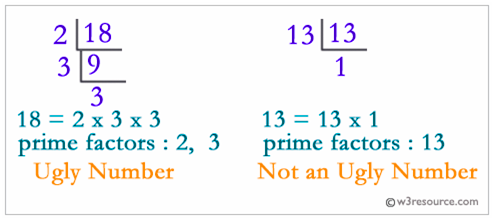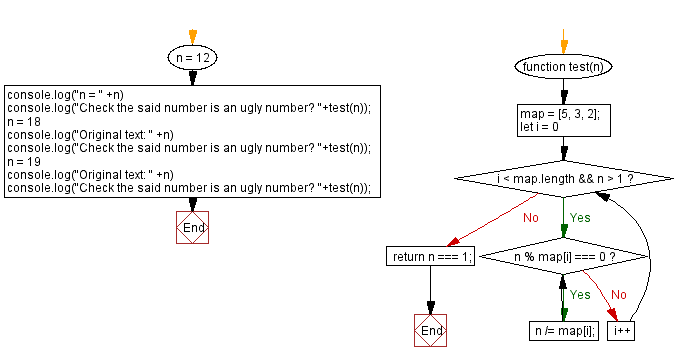# JavaScript: Check a given number is an ugly number or not

## JavaScript Math: Exercise-100 with Solution

Write a JavaScript program to check if a given number is ugly.

Ugly numbers are positive numbers whose only prime factors are 2, 3 or 5. The first 10 ugly numbers are:
1, 2, 3, 4, 5, 6, 8, 9, 10, 12, ...
Note: 1 is typically treated as an ugly number.

Visualisation:Test Data:
(12) -> true
(18) -> true
(19) -> false

Sample Solution:

HTML Code:

``````<!DOCTYPE html>
<html>
<meta charset="utf-8">
<title>JavaScript program to Check a given number is an ugly number or not</title>
<body>

</body>
</html>
```
```

JavaScript Code:

``````function test(n) {
map = [5, 3, 2];
for (let i = 0; i < map.length && n > 1; i++) {
while (n % map[i] === 0) n /= map[i];
}
return n === 1;
};
n = 12
console.log("n = " +n)
console.log("Check the said number is an ugly number? "+test(n));
n = 18
console.log("Original text: " +n)
console.log("Check the said number is an ugly number? "+test(n));
n = 19
console.log("Original text: " +n)
console.log("Check the said number is an ugly number? "+test(n));
```
```

Sample Output:

```n = 12
Check the said number is an ugly number? true
Original text: 18
Check the said number is an ugly number? true
Original text: 19
Check the said number is an ugly number? false
```

Flowchart:Live Demo:

See the Pen javascript-math-exercise-100 by w3resource (@w3resource) on CodePen.

Improve this sample solution and post your code through Disqus

What is the difficulty level of this exercise?

Test your Programming skills with w3resource's quiz.

﻿